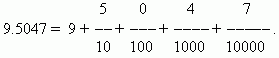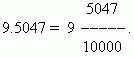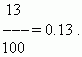# Decimal fractions (decimals)

Decimal fractions (decimal point, decimal places).
Properties of decimals. Repeating decimals.

Decimal fraction is a result of dividing of unit by ten, hundred, thousand parts etc. These fractions are very comfortable in calculations, because they are based on the same system, that calculus and record of integers are built. Due to this both record and rules of operations with decimal fractions are actually the same as for integers. At recording decimal fractions it isn’t necessary to mark parts  ( as denominator ); this is known by place, that the corresponding digit occupies. At first the integer part of a number is written; to the right of it the decimal point is put; the first digit after the point means a number of tenths ( a number of tenth parts of unit ), the second – a number of hundredths, the third – thousandths, and so on. Digits, located after decimal point, are called decimal places .

E x a m p l e .One of advantages of decimals – they are easily reduced to the shape of vulgar fractions: a number after a decimal point ( 5047 in our case ) is a numerator, and the n -th power of 10 ( n – a quantity of decimal places, in our case n = 4 ) is a denominator:If a decimal doesn’t contain an integer part, zero is put before a decimal point:Properties of decimals.

1. A decimal fraction isn’t changed, if to add some zeros to the right of it:

13.6 =13.6000.

2. A decimal fraction isn’t changed, if to reject zeros, located in the end:

0.00123000 = 0.00123 .

Note : it’s prohibited to reject zeros, located not in the end of a decimal !

 3 A decimal fraction will be increased by 10, 100, 1000 ,…times, if to transfer a decimal point to one, two, three, … places to the right:

3.675 ---> 367.5  (it increases by 100 times).

 4 A decimal fraction will be decreased by 10, 100, 1000 ,…times, if to transfer a decimal point to one, two, three, … places to the left:

1536.78 ---> 1.53678  (it decreases by 1000 times).

These properties permit quickly to multiply and to divide decimal fractions by 10, 100, 1000 and so on.

Repeating decimal is a decimal in which a digit or a group of digits repeats endlessly in a pattern. This group of repeating digits is called a period of decimal and is written in brackets. For instance,

E x a m p l e .   If to divide 47 by 11, then the result is 4.27272727… = 4.(27).